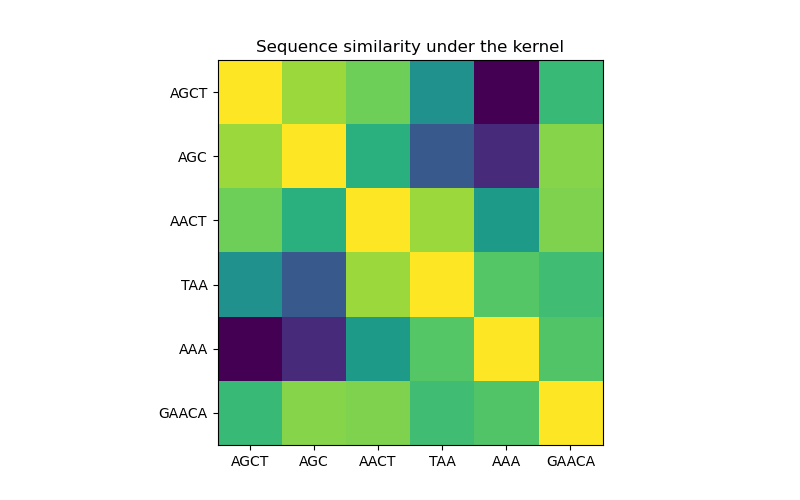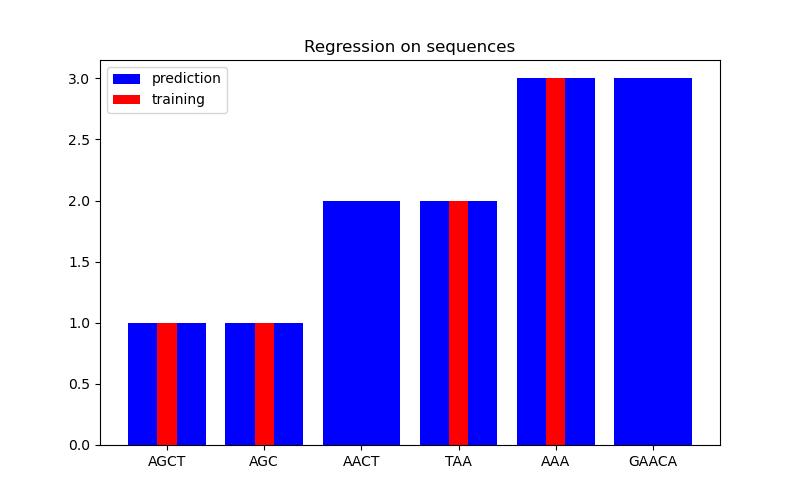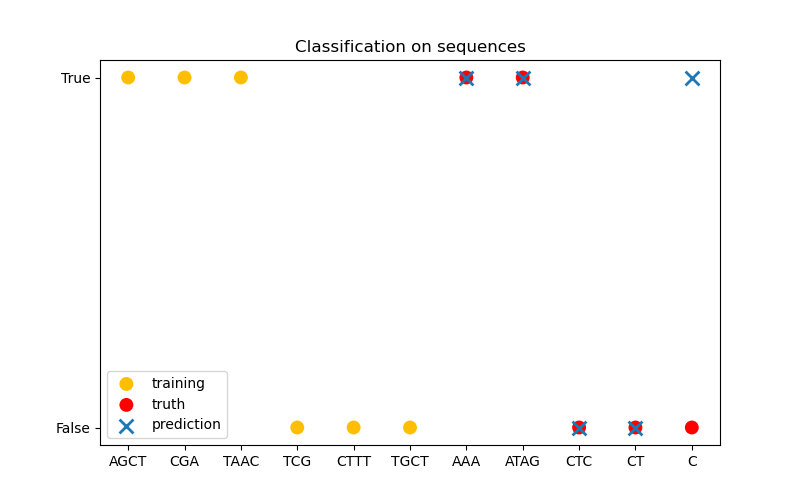# Gaussian processes on discrete data structures¶

This example illustrates the use of Gaussian processes for regression and classification tasks on data that are not in fixed-length feature vector form. This is achieved through the use of kernel functions that operates directly on discrete structures such as variable-length sequences, trees, and graphs.

Specifically, here the input variables are some gene sequences stored as variable-length strings consisting of letters ‘A’, ‘T’, ‘C’, and ‘G’, while the output variables are floating point numbers and True/False labels in the regression and classification tasks, respectively.

A kernel between the gene sequences is defined using R-convolution 1 by integrating a binary letter-wise kernel over all pairs of letters among a pair of strings.

This example will generate three figures.

In the first figure, we visualize the value of the kernel, i.e. the similarity of the sequences, using a colormap. Brighter color here indicates higher similarity.

In the second figure, we show some regression result on a dataset of 6 sequences. Here we use the 1st, 2nd, 4th, and 5th sequences as the training set to make predictions on the 3rd and 6th sequences.

In the third figure, we demonstrate a classification model by training on 6 sequences and make predictions on another 5 sequences. The ground truth here is simply whether there is at least one ‘A’ in the sequence. Here the model makes four correct classifications and fails on one.

1

Haussler, D. (1999). Convolution kernels on discrete structures (Vol. 646). Technical report, Department of Computer Science, University of California at Santa Cruz.

•••print(__doc__)

import numpy as np
import matplotlib.pyplot as plt
from sklearn.gaussian_process.kernels import Kernel, Hyperparameter
from sklearn.gaussian_process.kernels import GenericKernelMixin
from sklearn.gaussian_process import GaussianProcessRegressor
from sklearn.gaussian_process import GaussianProcessClassifier
from sklearn.base import clone

class SequenceKernel(GenericKernelMixin, Kernel):
'''
A minimal (but valid) convolutional kernel for sequences of variable
lengths.'''
def __init__(self,
baseline_similarity=0.5,
baseline_similarity_bounds=(1e-5, 1)):
self.baseline_similarity = baseline_similarity
self.baseline_similarity_bounds = baseline_similarity_bounds

@property
def hyperparameter_baseline_similarity(self):
return Hyperparameter("baseline_similarity",
"numeric",
self.baseline_similarity_bounds)

def _f(self, s1, s2):
'''
kernel value between a pair of sequences
'''
return sum([1.0 if c1 == c2 else self.baseline_similarity
for c1 in s1
for c2 in s2])

def _g(self, s1, s2):
'''
kernel derivative between a pair of sequences
'''
return sum([0.0 if c1 == c2 else 1.0
for c1 in s1
for c2 in s2])

if Y is None:
Y = X

return (np.array([[self._f(x, y) for y in Y] for x in X]),
np.array([[[self._g(x, y)] for y in Y] for x in X]))
else:
return np.array([[self._f(x, y) for y in Y] for x in X])

def diag(self, X):
return np.array([self._f(x, x) for x in X])

def is_stationary(self):
return False

def clone_with_theta(self, theta):
cloned = clone(self)
cloned.theta = theta
return cloned

kernel = SequenceKernel()

'''
Sequence similarity matrix under the kernel
===========================================
'''

X = np.array(['AGCT', 'AGC', 'AACT', 'TAA', 'AAA', 'GAACA'])

K = kernel(X)
D = kernel.diag(X)

plt.figure(figsize=(8, 5))
plt.imshow(np.diag(D**-0.5).dot(K).dot(np.diag(D**-0.5)))
plt.xticks(np.arange(len(X)), X)
plt.yticks(np.arange(len(X)), X)
plt.title('Sequence similarity under the kernel')

'''
Regression
==========
'''

X = np.array(['AGCT', 'AGC', 'AACT', 'TAA', 'AAA', 'GAACA'])
Y = np.array([1.0, 1.0, 2.0, 2.0, 3.0, 3.0])

training_idx = [0, 1, 3, 4]
gp = GaussianProcessRegressor(kernel=kernel)
gp.fit(X[training_idx], Y[training_idx])

plt.figure(figsize=(8, 5))
plt.bar(np.arange(len(X)), gp.predict(X), color='b', label='prediction')
plt.bar(training_idx, Y[training_idx], width=0.2, color='r',
alpha=1, label='training')
plt.xticks(np.arange(len(X)), X)
plt.title('Regression on sequences')
plt.legend()

'''
Classification
==============
'''

X_train = np.array(['AGCT', 'CGA', 'TAAC', 'TCG', 'CTTT', 'TGCT'])
# whether there are 'A's in the sequence
Y_train = np.array([True, True, True, False, False, False])

gp = GaussianProcessClassifier(kernel)
gp.fit(X_train, Y_train)

X_test = ['AAA', 'ATAG', 'CTC', 'CT', 'C']
Y_test = [True, True, False, False, False]

plt.figure(figsize=(8, 5))
plt.scatter(np.arange(len(X_train)), [1.0 if c else -1.0 for c in Y_train],
s=100, marker='o', edgecolor='none', facecolor=(1, 0.75, 0),
label='training')
plt.scatter(len(X_train) + np.arange(len(X_test)),
[1.0 if c else -1.0 for c in Y_test],
s=100, marker='o', edgecolor='none', facecolor='r', label='truth')
plt.scatter(len(X_train) + np.arange(len(X_test)),
[1.0 if c else -1.0 for c in gp.predict(X_test)],
s=100, marker='x', edgecolor=(0, 1.0, 0.3), linewidth=2,
label='prediction')
plt.xticks(np.arange(len(X_train) + len(X_test)),
np.concatenate((X_train, X_test)))
plt.yticks([-1, 1], [False, True])
plt.title('Classification on sequences')
plt.legend()

plt.show()


Total running time of the script: ( 0 minutes 0.279 seconds)

Gallery generated by Sphinx-Gallery